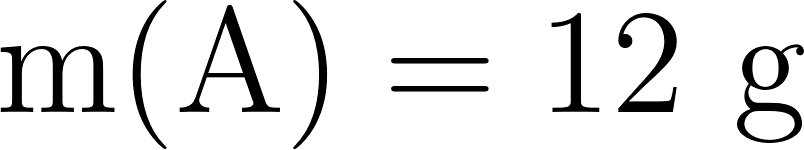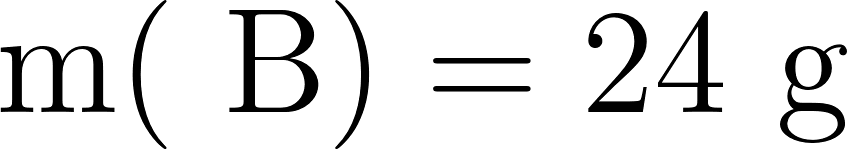### Solution Found!# Consider the generic chemical reaction:A + 2 B ? C + DSuppose you have 12 g of A and 24

Chapter 8, Problem 11Q

#### (choose chapter or problem)

Get Unlimited Answers! Check out our subscriptions

QUESTION:

Consider the generic chemical reaction:

A + 2 B  ⟶  C + D

Suppose you have 12 g of A and 24 g of B. Which statement is true?

(a) A will definitely be the limiting reactant.

(b) B will definitely be the limiting reactant.

(c) A will be the limiting reactant if its molar mass is less than B.

(d) A will be the limiting reactant if its molar mass is greater than B.

Step 1 of 3

Given reaction isAlso givenBecome a subscriber and get unlimited answers!